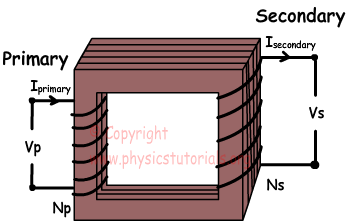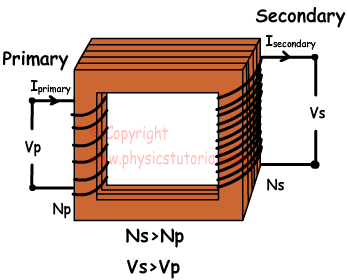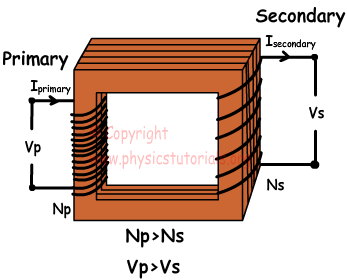## Transformers

Transformers

Transformers are devices used for changing the potential of alternating currents. Structure of simple transformer is given below;Voltage is applied to primary coil and, we take transformed voltage from secondary coil. There are two types of transformer, step up and step down. We use step down transformers in electrical devices like radio, and step up transformers in welding machine.

Step Up Transformer

This type of transformer used for increase the incident voltage. Number of turns in secondary coil is larger than the number of turns in primary coil.Step Down Transformer

This type of transformer used for decrease incident voltage. Number of turns in primary coil is larger than the number of turns in secondary coil.Transformer Equations

Vp is the potential, Ip is the current, Np is the turn on the primary coil and  Vs is the potential, Is is the current, Ns is the turn on the secondary coil. We use following equations to find potential, current or number of turns of any coil;

N1/N2=V1/V2=I2/I1

Magnetism Exams and Solutions

Author:

Tags: